moveit2 The MoveIt Motion Planning Framework for ROS 2.
moveit::core::JointModelGroup Class Reference

`#include <joint_model_group.h>`

Collaboration diagram for moveit::core::JointModelGroup: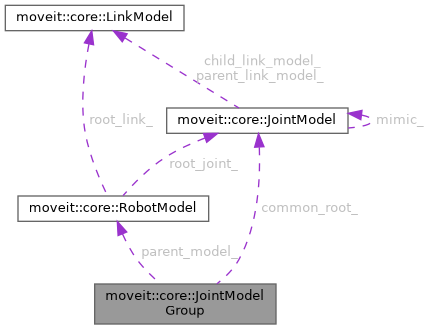[legend]

## Classes

struct  GroupMimicUpdate

struct  KinematicsSolver

## Public Types

using KinematicsSolverMap = std::map< const JointModelGroup *, KinematicsSolver >
Map from group instances to allocator functions & bijections. More...

## Public Member Functions

JointModelGroup (const std::string &name, const srdf::Model::Group &config, const std::vector< const JointModel * > &joint_vector, const RobotModel *parent_model)

~JointModelGroup ()

const RobotModelgetParentModel () const
Get the kinematic model this group is part of. More...

const std::string & getName () const
Get the name of the joint group. More...

const srdf::Model::Group & getConfig () const
get the SRDF configuration this group is based on More...

bool hasJointModel (const std::string &joint) const
Check if a joint is part of this group. More...

Check if a link is part of this group. More...

const JointModelgetJointModel (const std::string &joint) const
Get a joint by its name. Throw an exception if the joint is not part of this group. More...

Get a link by its name. Throw an exception if the link is not part of this group. More...

const std::vector< const JointModel * > & getJointModels () const
Get all the joints in this group (including fixed and mimic joints). More...

const std::vector< std::string > & getJointModelNames () const
Get the names of the joints in this group. These are the names of the joints returned by getJointModels(). More...

const std::vector< const JointModel * > & getActiveJointModels () const
Get the active joints in this group (that have controllable DOF). This does not include mimic joints. More...

const std::vector< std::string > & getActiveJointModelNames () const
Get the names of the active joints in this group. These are the names of the joints returned by getJointModels(). More...

const std::vector< const JointModel * > & getFixedJointModels () const
Get the fixed joints that are part of this group. More...

const std::vector< const JointModel * > & getMimicJointModels () const
Get the mimic joints that are part of this group. More...

const std::vector< const JointModel * > & getContinuousJointModels () const
Get the array of continuous joints used in this group (may include mimic joints). More...

const std::vector< std::string > & getVariableNames () const
Get the names of the variables that make up the joints included in this group. The number of returned elements is always equal to getVariableCount(). This includes mimic joints. More...

const std::vector< const JointModel * > & getJointRoots () const
Unlike a complete kinematic model, a group may contain disconnected parts of the kinematic tree – a set of smaller trees. This function gives the roots of those smaller trees. Furthermore, it is ensured that the roots are on different branches in the kinematic tree. This means that in following any root in the given list, none of the other returned roots will be encountered. More...

const JointModelgetCommonRoot () const
Get the common root of all joint roots; not necessarily part of this group. More...

Get the links that are part of this joint group. More...

const std::vector< std::string > & getLinkModelNames () const
Get the names of the links that are part of this joint group. More...

const std::vector< std::string > & getLinkModelNamesWithCollisionGeometry () const
Get the names of the links that are part of this joint group and also have geometry associated with them. More...

Get the names of the links that are to be updated when the state of this group changes. This includes links that are in the kinematic model but outside this group, if those links are descendants of joints in this group that have their values updated. The order is the correct order for updating the corresponding states. More...

Return the same data as getUpdatedLinkModels() but as a set. More...

const std::vector< std::string > & getUpdatedLinkModelNames () const

Get the names of the links that are to be updated when the state of this group changes. This includes links that are in the kinematic model but outside this group, if those links are descendants of joints in this group that have their values updated. More...

Return the same data as getUpdatedLinkModelsWithGeometry() but as a set. More...

const std::vector< std::string > & getUpdatedLinkModelsWithGeometryNames () const

const std::set< std::string > & getUpdatedLinkModelsWithGeometryNamesSet () const

bool isLinkUpdated (const std::string &name) const
True if this name is in the set of links that are to be updated when the state of this group changes. This includes links that are in the kinematic model but outside this group, if those links are descendants of joints in this group that have their values updated. More...

const std::vector< int > & getVariableIndexList () const
Get the index locations in the complete robot state for all the variables in this group. More...

int getVariableGroupIndex (const std::string &variable) const
Get the index of a variable within the group. Return -1 on error. More...

const std::vector< std::string > & getDefaultStateNames () const
Get the names of the known default states (as specified in the SRDF) More...

void addDefaultState (const std::string &name, const std::map< std::string, double > &default_state)

bool getVariableDefaultPositions (const std::string &name, std::map< std::string, double > &values) const
Get the values that correspond to a named state as read from the URDF. Return false on failure. More...

void getVariableDefaultPositions (std::map< std::string, double > &values) const
Compute the default values for the joint group. More...

void getVariableDefaultPositions (std::vector< double > &values) const
Compute the default values for the joint group. More...

void getVariableDefaultPositions (double *values) const
Compute the default values for the joint group. More...

void getVariableRandomPositions (random_numbers::RandomNumberGenerator &rng, double *values) const
Compute random values for the state of the joint group. More...

void getVariableRandomPositions (random_numbers::RandomNumberGenerator &rng, std::vector< double > &values) const
Compute random values for the state of the joint group. More...

void getVariableRandomPositionsNearBy (random_numbers::RandomNumberGenerator &rng, double *values, const double *near, const double distance) const
Compute random values for the state of the joint group. More...

void getVariableRandomPositionsNearBy (random_numbers::RandomNumberGenerator &rng, std::vector< double > &values, const std::vector< double > &near, double distance) const
Compute random values for the state of the joint group. More...

void getVariableRandomPositionsNearBy (random_numbers::RandomNumberGenerator &rng, std::vector< double > &values, const std::vector< double > &near, const std::map< JointModel::JointType, double > &distance_map) const
Compute random values for the state of the joint group. More...

void getVariableRandomPositionsNearBy (random_numbers::RandomNumberGenerator &rng, double *values, const double *near, const std::vector< double > &distances) const
Compute random values for the state of the joint group. More...

void getVariableRandomPositionsNearBy (random_numbers::RandomNumberGenerator &rng, std::vector< double > &values, const std::vector< double > &near, const std::vector< double > &distances) const
Compute random values for the state of the joint group. More...

void getVariableRandomPositions (random_numbers::RandomNumberGenerator &rng, double *values, const JointBoundsVector &active_joint_bounds) const

void getVariableRandomPositionsNearBy (random_numbers::RandomNumberGenerator &rng, double *values, const JointBoundsVector &active_joint_bounds, const double *near, const double distance) const
Compute random values for the state of the joint group. More...

void getVariableRandomPositionsNearBy (random_numbers::RandomNumberGenerator &rng, double *values, const JointBoundsVector &active_joint_bounds, const double *near, const std::map< JointModel::JointType, double > &distance_map) const
Compute random values for the state of the joint group. More...

void getVariableRandomPositionsNearBy (random_numbers::RandomNumberGenerator &rng, double *values, const JointBoundsVector &active_joint_bounds, const double *near, const std::vector< double > &distances) const
Compute random values for the state of the joint group. More...

bool enforcePositionBounds (double *state) const

bool enforcePositionBounds (double *state, const JointBoundsVector &active_joint_bounds) const

bool satisfiesPositionBounds (const double *state, double margin=0.0) const

bool satisfiesPositionBounds (const double *state, const JointBoundsVector &active_joint_bounds, double margin=0.0) const

double getMaximumExtent () const

double getMaximumExtent (const JointBoundsVector &active_joint_bounds) const

double distance (const double *state1, const double *state2) const

void interpolate (const double *from, const double *to, double t, double *state) const

unsigned int getVariableCount () const
Get the number of variables that describe this joint group. This includes variables necessary for mimic joints, so will always be >= the number of items returned by getActiveVariableNames() More...

unsigned int getActiveVariableCount () const
Get the number of variables that describe the active joints in this joint group. This excludes variables necessary for mimic joints. More...

void setSubgroupNames (const std::vector< std::string > &subgroups)
Set the names of the subgroups for this group. More...

const std::vector< std::string > & getSubgroupNames () const
Get the names of the groups that are subsets of this one (in terms of joints set) More...

void getSubgroups (std::vector< const JointModelGroup * > &sub_groups) const
Get the groups that are subsets of this one (in terms of joints set) More...

bool isSubgroup (const std::string &group) const
Check if the joints of group group are a subset of the joints in this group. More...

bool isChain () const
Check if this group is a linear chain. More...

bool isSingleDOFJoints () const
Return true if the group consists only of joints that are single DOF. More...

bool isEndEffector () const
Check if this group was designated as an end-effector in the SRDF. More...

bool isContiguousWithinState () const

const std::string & getEndEffectorName () const
Return the name of the end effector, if this group is an end-effector. More...

void setEndEffectorName (const std::string &name)
Set the name of the end-effector, and remember this group is indeed an end-effector. More...

void setEndEffectorParent (const std::string &group, const std::string &link)
If this group is an end-effector, specify the parent group (e.g., the arm holding the eef) and the link the end effector connects to. More...

void attachEndEffector (const std::string &eef_name)
Notify this group that there is an end-effector attached to it. More...

const std::pair< std::string, std::string > & getEndEffectorParentGroup () const
Get the name of the group this end-effector attaches to (first) and the name of the link in that group (second) More...

const std::vector< std::string > & getAttachedEndEffectorNames () const
Get the names of the end effectors attached to this group. More...

bool getEndEffectorTips (std::vector< const LinkModel * > &tips) const
Get the unique set of end effector tips included in a particular joint model group as defined by the SRDF end effector elements e.g. for a humanoid robot this would return 4 tips for the hands and feet. More...

bool getEndEffectorTips (std::vector< std::string > &tips) const
Get the unique set of end effector tips included in a particular joint model group as defined by the SRDF end effector elements e.g. for a humanoid robot this would return 4 tips for the hands and feet. More...

Get one end effector tip, throwing an error if there ends up being more in the joint model group This is a useful helper function because most planning groups (almost all) only have one tip. More...

const JointBoundsVectorgetActiveJointModelsBounds () const
Get the bounds for all the active joints. More...

const std::pair< KinematicsSolver, KinematicsSolverMap > & getGroupKinematics () const

void setSolverAllocators (const SolverAllocatorFn &solver, const SolverAllocatorMapFn &solver_map=SolverAllocatorMapFn())

void setSolverAllocators (const std::pair< SolverAllocatorFn, SolverAllocatorMapFn > &solvers)

const kinematics::KinematicsBaseConstPtr getSolverInstance () const

const kinematics::KinematicsBasePtr & getSolverInstance ()

bool canSetStateFromIK (const std::string &tip) const

bool setRedundantJoints (const std::vector< std::string > &joints)

double getDefaultIKTimeout () const
Get the default IK timeout. More...

void setDefaultIKTimeout (double ik_timeout)
Set the default IK timeout. More...

const std::vector< size_t > & getKinematicsSolverJointBijection () const
Return the mapping between the order of the joints in this group and the order of the joints in the kinematics solver. An element bijection[i] at index i in this array, maps the variable at index bijection[i] in this group to the variable at index i in the kinematic solver. More...

void printGroupInfo (std::ostream &out=std::cout) const
Print information about the constructed model. More...

bool isValidVelocityMove (const std::vector< double > &from_joint_pose, const std::vector< double > &to_joint_pose, double dt) const
Check that the time to move between two waypoints is sufficient given velocity limits. More...

bool isValidVelocityMove (const double *from_joint_pose, const double *to_joint_pose, std::size_t array_size, double dt) const
Check that the time to move between two waypoints is sufficient given velocity limits. More...

bool computeJointVariableIndices (const std::vector< std::string > &joint_names, std::vector< size_t > &joint_bijection) const
Computes the indices of joint variables given a vector of joint names to look up. More...

## Protected Member Functions

void updateMimicJoints (double *values) const
Update the variable values for the state of a group with respect to the mimic joints. This only updates mimic joints that have the parent in this group. If there is a joint mimicking one that is outside the group, there are no values to be read (values is only the group state) More...

## Protected Attributes

const RobotModelparent_model_
Owner model. More...

std::string name_
Name of group. More...

std::vector< const JointModel * > joint_model_vector_
Joint instances in the order they appear in the group state. More...

std::vector< std::string > joint_model_name_vector_
Names of joints in the order they appear in the group state. More...

std::vector< const JointModel * > active_joint_model_vector_
Active joint instances in the order they appear in the group state. More...

std::vector< std::string > active_joint_model_name_vector_
Names of active joints in the order they appear in the group state. More...

std::vector< const JointModel * > fixed_joints_
The joints that have no DOF (fixed) More...

std::vector< const JointModel * > mimic_joints_
Joints that mimic other joints. More...

std::vector< const JointModel * > continuous_joint_model_vector_
The set of continuous joints this group contains. More...

std::vector< std::string > variable_names_
The names of the DOF that make up this group (this is just a sequence of joint variable names; not necessarily joint names!) More...

std::set< std::string > variable_names_set_
The names of the DOF that make up this group (this is just a sequence of joint variable names; not necessarily joint names!) More...

JointModelMapConst joint_model_map_
A map from joint names to their instances. This includes all joints in the group. More...

std::vector< const JointModel * > joint_roots_
The list of active joint models that are roots in this group. More...

const JointModelcommon_root_
The joint that is a common root for all joints in this group (not necessarily part of this group) More...

VariableIndexMap joint_variables_index_map_
The group includes all the joint variables that make up the joints the group consists of. This map gives the position in the state vector of the group for each of these variables. Additionally, it includes the names of the joints and the index for the first variable of that joint. More...

JointBoundsVector active_joint_models_bounds_
The bounds for all the active joint models. More...

std::vector< int > variable_index_list_
The list of index values this group includes, with respect to a full robot state; this includes mimic joints. More...

std::vector< int > active_joint_model_start_index_
For each active joint model in this group, hold the index at which the corresponding joint state starts in the group state. More...

The links that are on the direct lineage between joints and joint_roots_, as well as the children of the joint leafs. May not be in any particular order. More...

A map from link names to their instances. More...

The names of the links in this group. More...

The names of the links in this group that also have geometry. More...

The list of downstream link models in the order they should be updated (may include links that are not in this group) More...

The list of downstream link models in the order they should be updated (may include links that are not in this group) More...

The list of downstream link names in the order they should be updated (may include links that are not in this group) More...

The list of downstream link names in the order they should be updated (may include links that are not in this group) More...

The list of downstream link models in the order they should be updated (may include links that are not in this group) More...

The list of downstream link models in the order they should be updated (may include links that are not in this group) More...

The list of downstream link names in the order they should be updated (may include links that are not in this group) More...

The list of downstream link names in the order they should be updated (may include links that are not in this group) More...

unsigned int variable_count_
The number of variables necessary to describe this group of joints. More...

unsigned int active_variable_count_
The number of variables necessary to describe the active joints in this group of joints. More...

bool is_contiguous_index_list_
True if the state of this group is contiguous within the full robot state; this also means that the index values in variable_index_list_ are consecutive integers. More...

std::vector< std::string > subgroup_names_
The set of labelled subgroups that are included in this group. More...

std::set< std::string > subgroup_names_set_
The set of labelled subgroups that are included in this group. More...

std::vector< std::string > attached_end_effector_names_
If an end-effector is attached to this group, the name of that end-effector is stored in this variable. More...

std::pair< std::string, std::string > end_effector_parent_
First: name of the group that is parent to this end-effector group; Second: the link this in the parent group that this group attaches to. More...

std::string end_effector_name_
The name of the end effector, if this group is an end-effector. More...

bool is_chain_

bool is_single_dof_

std::vector< GroupMimicUpdategroup_mimic_update_

std::pair< KinematicsSolver, KinematicsSolverMapgroup_kinematics_

srdf::Model::Group config_

std::map< std::string, std::map< std::string, double > > default_states_
The set of default states specified for this group in the SRDF. More...

std::vector< std::string > default_states_names_
The names of the default states specified for this group in the SRDF. More...

## Detailed Description

Definition at line 69 of file joint_model_group.h.

## ◆ KinematicsSolverMap

 using moveit::core::JointModelGroup::KinematicsSolverMap = std::map

Map from group instances to allocator functions & bijections.

Definition at line 106 of file joint_model_group.h.

## ◆ JointModelGroup()

 moveit::core::JointModelGroup::JointModelGroup ( const std::string & name, const srdf::Model::Group & config, const std::vector< const JointModel * > & joint_vector, const RobotModel * parent_model )

Definition at line 111 of file joint_model_group.cpp.

Here is the call graph for this function: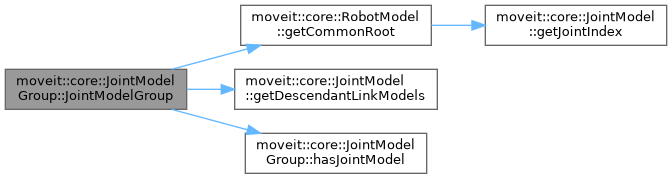## ◆ ~JointModelGroup()

 moveit::core::JointModelGroup::~JointModelGroup ( )
default

## Member Function Documentation

 void moveit::core::JointModelGroup::addDefaultState ( const std::string & name, const std::map< std::string, double > & default_state )

Definition at line 483 of file joint_model_group.cpp.

## ◆ attachEndEffector()

 void moveit::core::JointModelGroup::attachEndEffector ( const std::string & eef_name )

Notify this group that there is an end-effector attached to it.

Definition at line 524 of file joint_model_group.cpp.

## ◆ canSetStateFromIK()

 bool moveit::core::JointModelGroup::canSetStateFromIK ( const std::string & tip ) const

Definition at line 669 of file joint_model_group.cpp.

Here is the call graph for this function: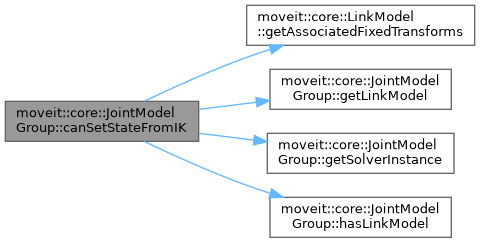## ◆ computeJointVariableIndices()

 bool moveit::core::JointModelGroup::computeJointVariableIndices ( const std::vector< std::string > & joint_names, std::vector< size_t > & joint_bijection ) const

Computes the indices of joint variables given a vector of joint names to look up.

Definition at line 609 of file joint_model_group.cpp.

Here is the call graph for this function: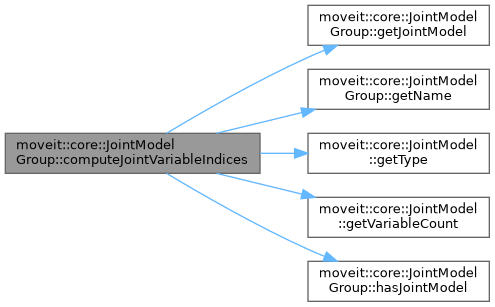Here is the caller graph for this function: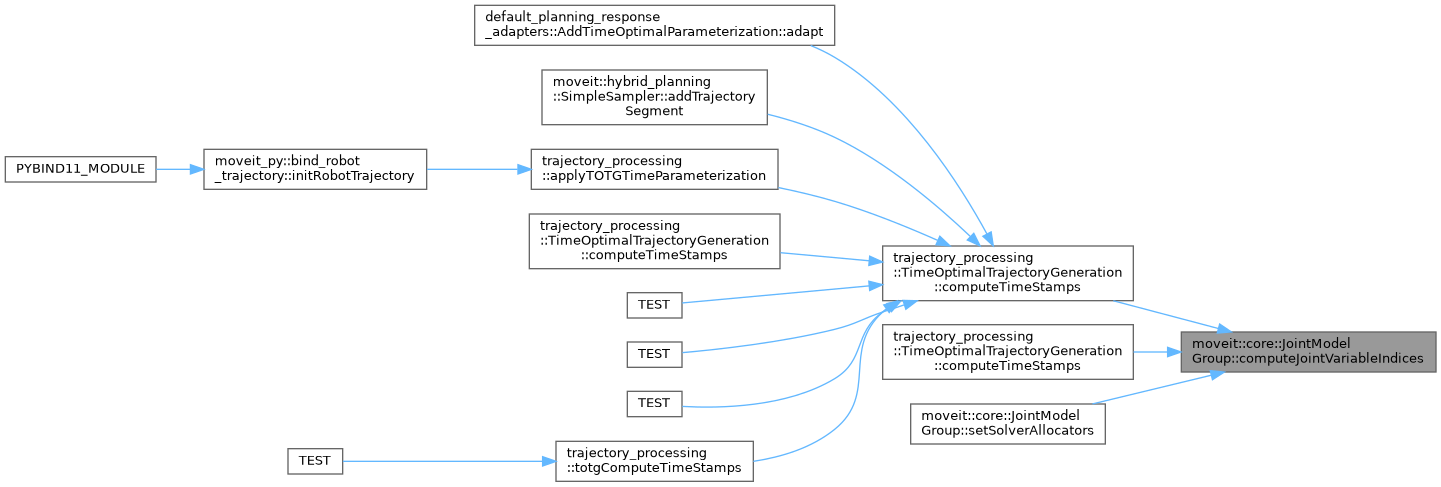## ◆ distance()

 double moveit::core::JointModelGroup::distance ( const double * state1, const double * state2 ) const

Definition at line 450 of file joint_model_group.cpp.

Here is the caller graph for this function: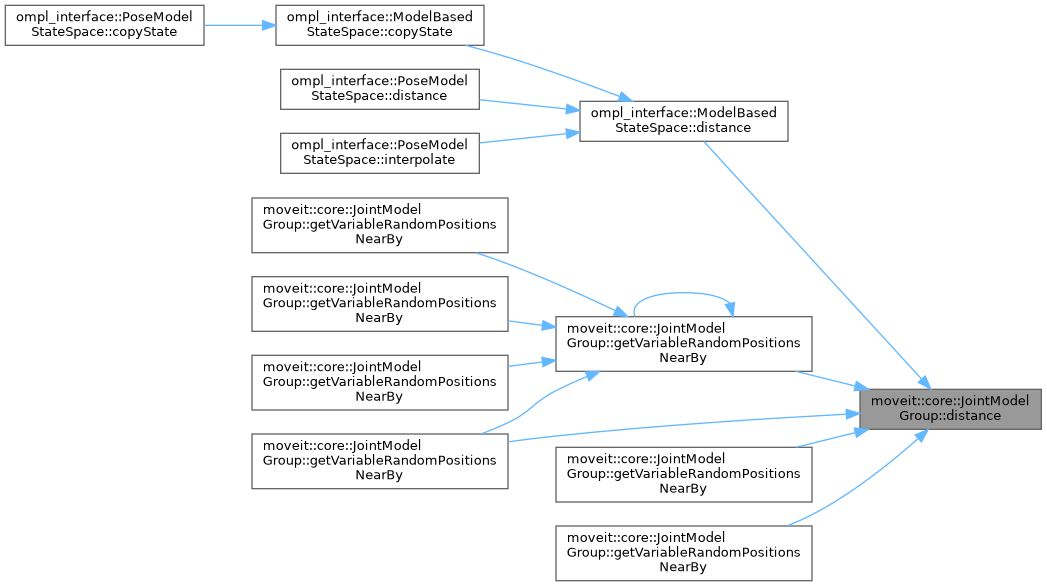## ◆ enforcePositionBounds() [1/2]

 bool moveit::core::JointModelGroup::enforcePositionBounds ( double * state ) const
inline

Definition at line 386 of file joint_model_group.h.

Here is the caller graph for this function: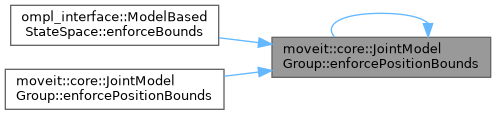## ◆ enforcePositionBounds() [2/2]

 bool moveit::core::JointModelGroup::enforcePositionBounds ( double * state, const JointBoundsVector & active_joint_bounds ) const

Definition at line 424 of file joint_model_group.cpp.

Here is the call graph for this function: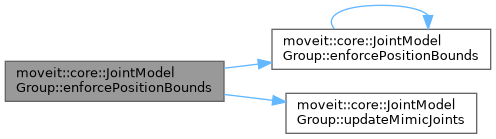## ◆ getActiveJointModelNames()

 const std::vector& moveit::core::JointModelGroup::getActiveJointModelNames ( ) const
inline

Get the names of the active joints in this group. These are the names of the joints returned by getJointModels().

Definition at line 164 of file joint_model_group.h.

Here is the caller graph for this function:## ◆ getActiveJointModels()

 const std::vector& moveit::core::JointModelGroup::getActiveJointModels ( ) const
inline

Get the active joints in this group (that have controllable DOF). This does not include mimic joints.

Definition at line 157 of file joint_model_group.h.

Here is the caller graph for this function:## ◆ getActiveJointModelsBounds()

 const JointBoundsVector& moveit::core::JointModelGroup::getActiveJointModelsBounds ( ) const
inline

Get the bounds for all the active joints.

Definition at line 519 of file joint_model_group.h.

Here is the caller graph for this function:## ◆ getActiveVariableCount()

 unsigned int moveit::core::JointModelGroup::getActiveVariableCount ( ) const
inline

Get the number of variables that describe the active joints in this joint group. This excludes variables necessary for mimic joints.

Definition at line 417 of file joint_model_group.h.

Here is the caller graph for this function: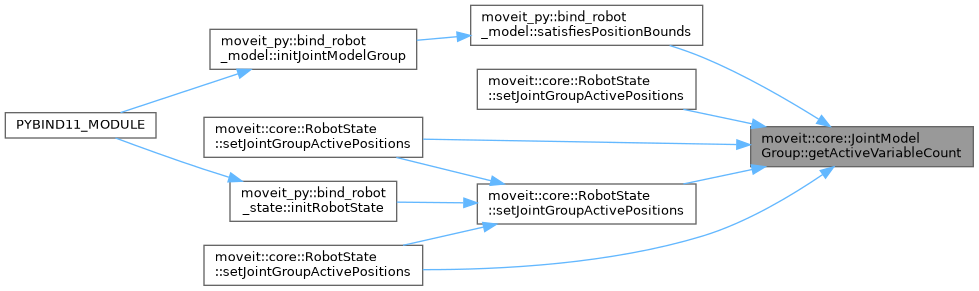## ◆ getAttachedEndEffectorNames()

 const std::vector& moveit::core::JointModelGroup::getAttachedEndEffectorNames ( ) const
inline

Get the names of the end effectors attached to this group.

Definition at line 488 of file joint_model_group.h.

Here is the caller graph for this function:## ◆ getCommonRoot()

 const JointModel* moveit::core::JointModelGroup::getCommonRoot ( ) const
inline

Get the common root of all joint roots; not necessarily part of this group.

Definition at line 207 of file joint_model_group.h.

## ◆ getConfig()

 const srdf::Model::Group& moveit::core::JointModelGroup::getConfig ( ) const
inline

get the SRDF configuration this group is based on

Definition at line 126 of file joint_model_group.h.

## ◆ getContinuousJointModels()

 const std::vector& moveit::core::JointModelGroup::getContinuousJointModels ( ) const
inline

Get the array of continuous joints used in this group (may include mimic joints).

Definition at line 182 of file joint_model_group.h.

Here is the caller graph for this function:## ◆ getDefaultIKTimeout()

 double moveit::core::JointModelGroup::getDefaultIKTimeout ( ) const
inline

Get the default IK timeout.

Definition at line 557 of file joint_model_group.h.

Here is the caller graph for this function: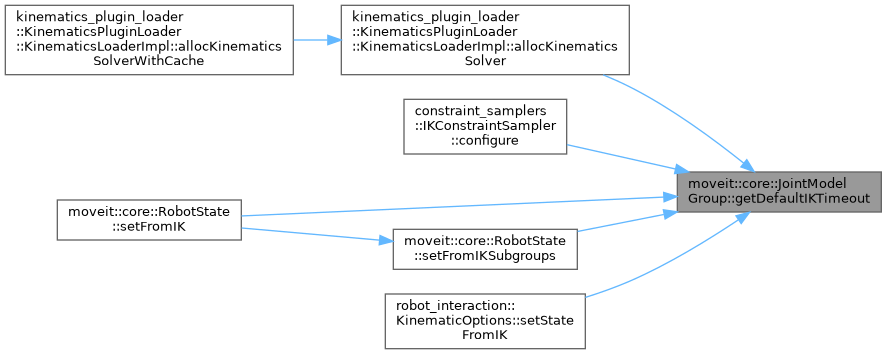## ◆ getDefaultStateNames()

 const std::vector& moveit::core::JointModelGroup::getDefaultStateNames ( ) const
inline

Get the names of the known default states (as specified in the SRDF)

Definition at line 295 of file joint_model_group.h.

Here is the caller graph for this function:## ◆ getEndEffectorName()

 const std::string& moveit::core::JointModelGroup::getEndEffectorName ( ) const
inline

Return the name of the end effector, if this group is an end-effector.

Definition at line 464 of file joint_model_group.h.

## ◆ getEndEffectorParentGroup()

 const std::pair& moveit::core::JointModelGroup::getEndEffectorParentGroup ( ) const
inline

Get the name of the group this end-effector attaches to (first) and the name of the link in that group (second)

Definition at line 482 of file joint_model_group.h.

Here is the caller graph for this function:## ◆ getEndEffectorTips() [1/2]

 bool moveit::core::JointModelGroup::getEndEffectorTips ( std::vector< const LinkModel * > & tips ) const

Get the unique set of end effector tips included in a particular joint model group as defined by the SRDF end effector elements e.g. for a humanoid robot this would return 4 tips for the hands and feet.

Parameters
 tips - the output vector of link models of the tips
Returns
true on success

Definition at line 543 of file joint_model_group.cpp.

Here is the call graph for this function: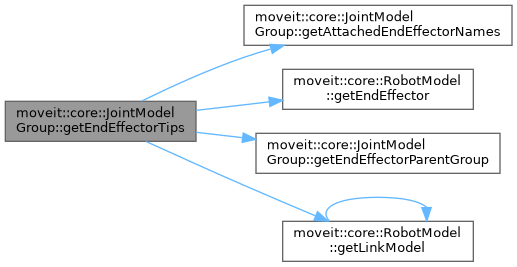Here is the caller graph for this function: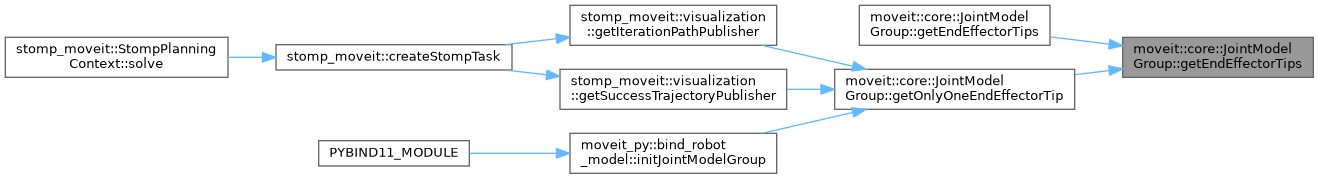## ◆ getEndEffectorTips() [2/2]

 bool moveit::core::JointModelGroup::getEndEffectorTips ( std::vector< std::string > & tips ) const

Get the unique set of end effector tips included in a particular joint model group as defined by the SRDF end effector elements e.g. for a humanoid robot this would return 4 tips for the hands and feet.

Parameters
 tips - the output vector of link names of the tips
Returns
true on success

Definition at line 529 of file joint_model_group.cpp.

Here is the call graph for this function: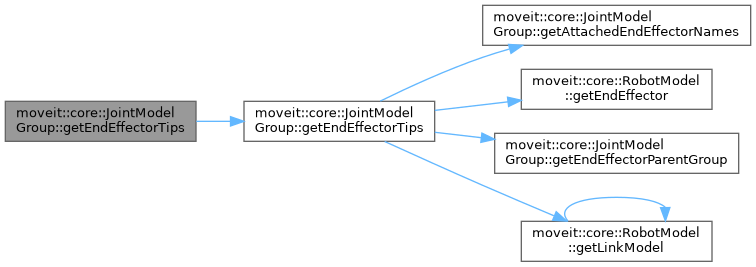## ◆ getFixedJointModels()

 const std::vector& moveit::core::JointModelGroup::getFixedJointModels ( ) const
inline

Get the fixed joints that are part of this group.

Definition at line 170 of file joint_model_group.h.

## ◆ getGroupKinematics()

 const std::pair& moveit::core::JointModelGroup::getGroupKinematics ( ) const
inline

Definition at line 524 of file joint_model_group.h.

Here is the caller graph for this function:## ◆ getJointModel()

 const JointModel * moveit::core::JointModelGroup::getJointModel ( const std::string & joint ) const

Get a joint by its name. Throw an exception if the joint is not part of this group.

Definition at line 325 of file joint_model_group.cpp.

Here is the caller graph for this function: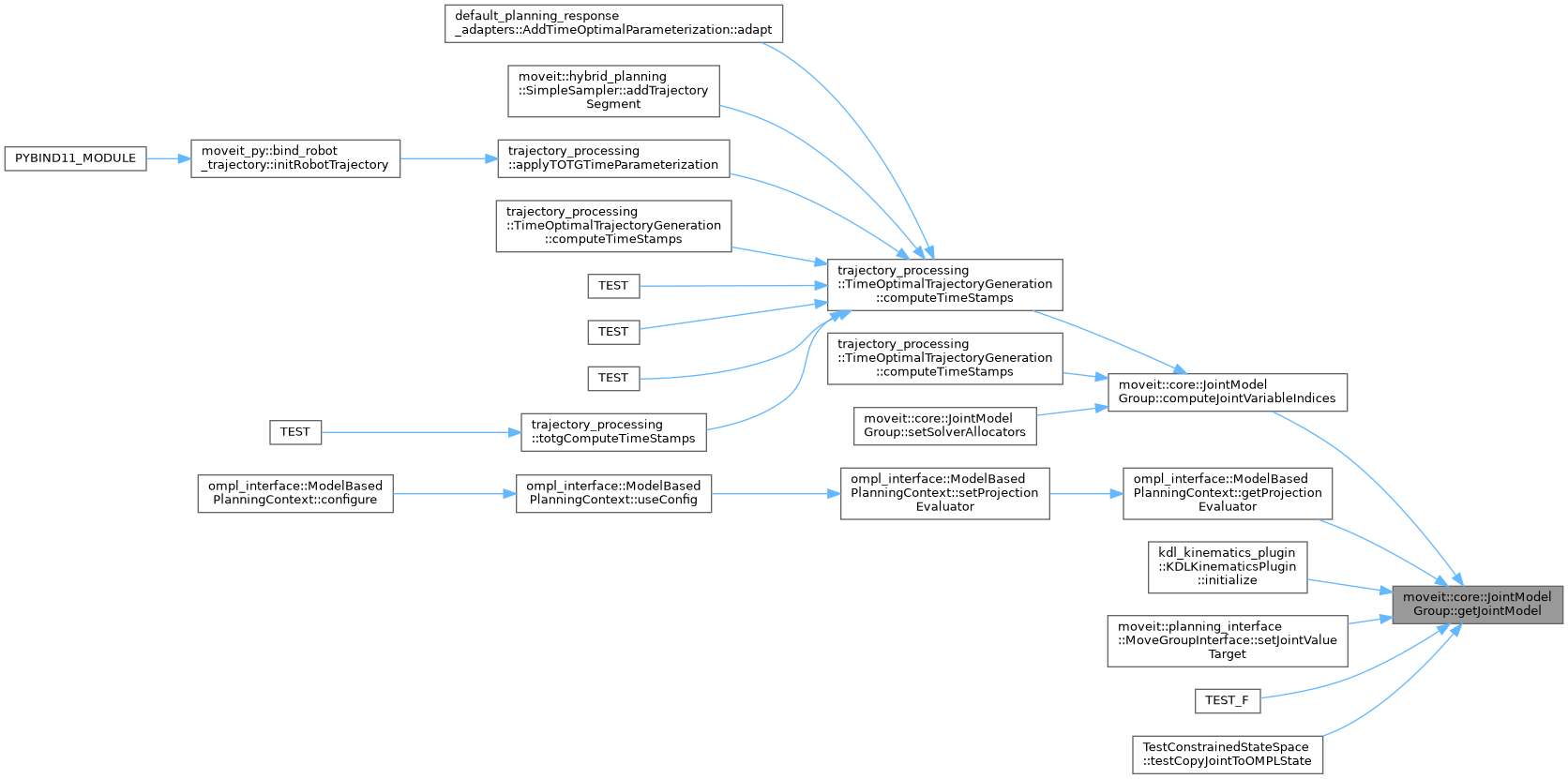## ◆ getJointModelNames()

 const std::vector& moveit::core::JointModelGroup::getJointModelNames ( ) const
inline

Get the names of the joints in this group. These are the names of the joints returned by getJointModels().

Definition at line 151 of file joint_model_group.h.

Here is the caller graph for this function:## ◆ getJointModels()

 const std::vector& moveit::core::JointModelGroup::getJointModels ( ) const
inline

Get all the joints in this group (including fixed and mimic joints).

Definition at line 144 of file joint_model_group.h.

Here is the caller graph for this function:## ◆ getJointRoots()

 const std::vector& moveit::core::JointModelGroup::getJointRoots ( ) const
inline

Unlike a complete kinematic model, a group may contain disconnected parts of the kinematic tree – a set of smaller trees. This function gives the roots of those smaller trees. Furthermore, it is ensured that the roots are on different branches in the kinematic tree. This means that in following any root in the given list, none of the other returned roots will be encountered.

Definition at line 201 of file joint_model_group.h.

Here is the caller graph for this function:## ◆ getKinematicsSolverJointBijection()

 const std::vector& moveit::core::JointModelGroup::getKinematicsSolverJointBijection ( ) const
inline

Return the mapping between the order of the joints in this group and the order of the joints in the kinematics solver. An element bijection[i] at index i in this array, maps the variable at index bijection[i] in this group to the variable at index i in the kinematic solver.

Definition at line 569 of file joint_model_group.h.

Here is the caller graph for this function:Get a link by its name. Throw an exception if the link is not part of this group.

Definition at line 314 of file joint_model_group.cpp.

Here is the caller graph for this function: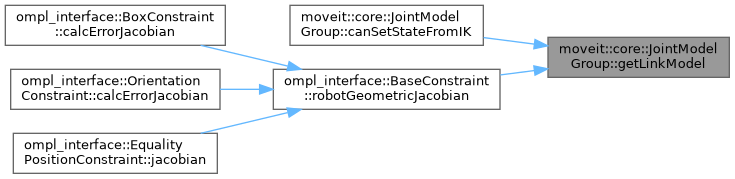const std::vector& moveit::core::JointModelGroup::getLinkModelNames ( ) const
inline

Get the names of the links that are part of this joint group.

Definition at line 219 of file joint_model_group.h.

Here is the caller graph for this function:const std::vector& moveit::core::JointModelGroup::getLinkModelNamesWithCollisionGeometry ( ) const
inline

Get the names of the links that are part of this joint group and also have geometry associated with them.

Definition at line 225 of file joint_model_group.h.

Here is the caller graph for this function:const std::vector& moveit::core::JointModelGroup::getLinkModels ( ) const
inline

Get the links that are part of this joint group.

Definition at line 213 of file joint_model_group.h.

Here is the caller graph for this function:## ◆ getMaximumExtent() [1/2]

 double moveit::core::JointModelGroup::getMaximumExtent ( ) const
inline

Definition at line 399 of file joint_model_group.h.

## ◆ getMaximumExtent() [2/2]

 double moveit::core::JointModelGroup::getMaximumExtent ( const JointBoundsVector & active_joint_bounds ) const

Definition at line 439 of file joint_model_group.cpp.

## ◆ getMimicJointModels()

 const std::vector& moveit::core::JointModelGroup::getMimicJointModels ( ) const
inline

Get the mimic joints that are part of this group.

Definition at line 176 of file joint_model_group.h.

Here is the caller graph for this function:## ◆ getName()

 const std::string& moveit::core::JointModelGroup::getName ( ) const
inline

Get the name of the joint group.

Definition at line 120 of file joint_model_group.h.

Here is the caller graph for this function:## ◆ getOnlyOneEndEffectorTip()

 const LinkModel * moveit::core::JointModelGroup::getOnlyOneEndEffectorTip ( ) const

Get one end effector tip, throwing an error if there ends up being more in the joint model group This is a useful helper function because most planning groups (almost all) only have one tip.

Returns
pointer to LinkModel, or nullptr on failure

Definition at line 570 of file joint_model_group.cpp.

Here is the call graph for this function: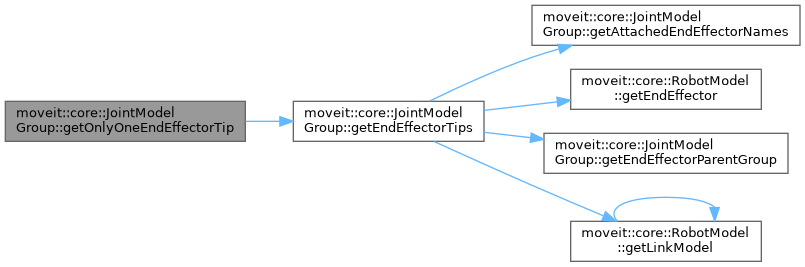## ◆ getParentModel()

 const RobotModel& moveit::core::JointModelGroup::getParentModel ( ) const
inline

Get the kinematic model this group is part of.

Definition at line 114 of file joint_model_group.h.

Here is the caller graph for this function:## ◆ getSolverInstance() [1/2]

 const kinematics::KinematicsBasePtr& moveit::core::JointModelGroup::getSolverInstance ( )
inline

Definition at line 542 of file joint_model_group.h.

## ◆ getSolverInstance() [2/2]

 const kinematics::KinematicsBaseConstPtr moveit::core::JointModelGroup::getSolverInstance ( ) const
inline

Definition at line 537 of file joint_model_group.h.

Here is the caller graph for this function: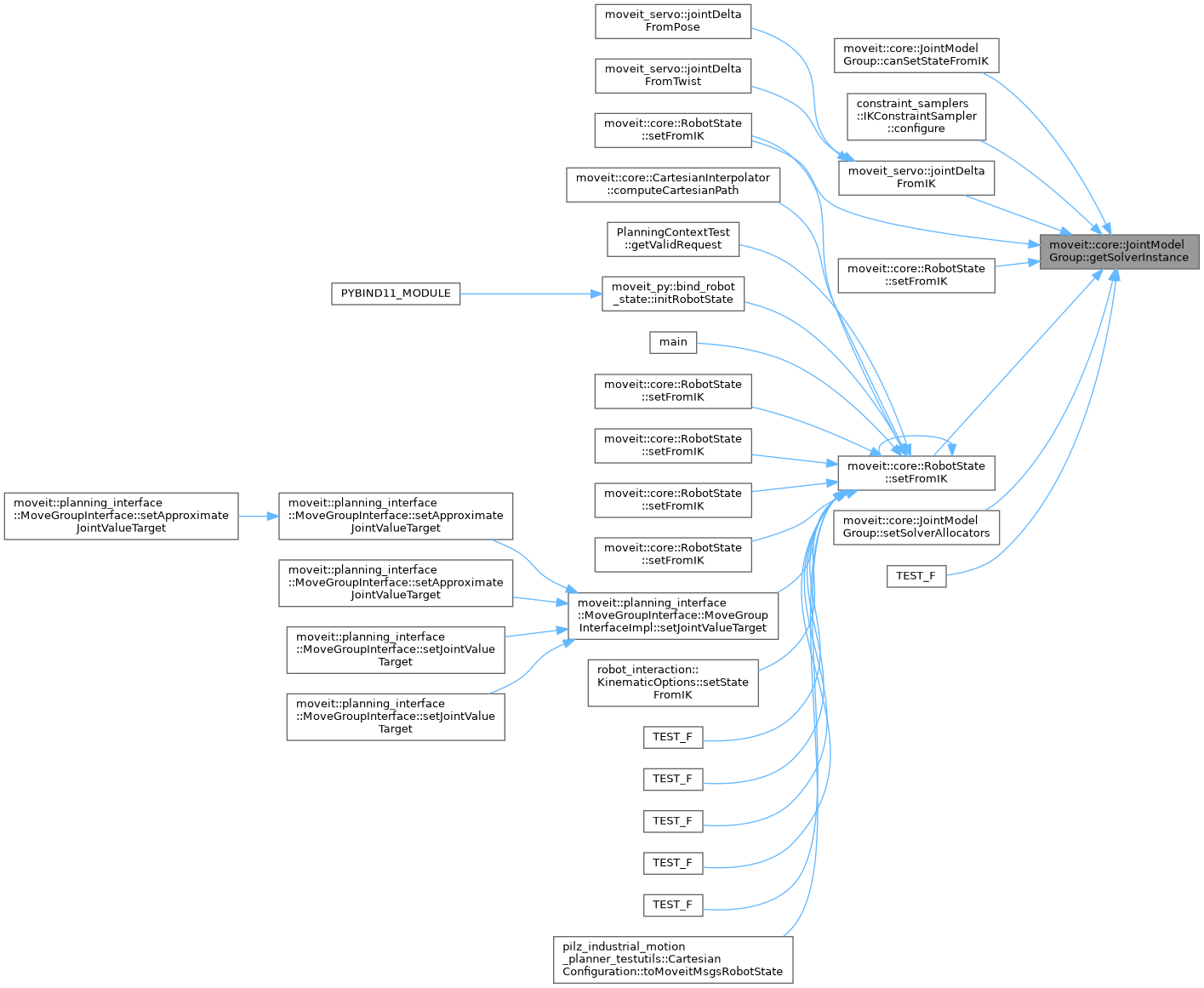## ◆ getSubgroupNames()

 const std::vector& moveit::core::JointModelGroup::getSubgroupNames ( ) const
inline

Get the names of the groups that are subsets of this one (in terms of joints set)

Definition at line 426 of file joint_model_group.h.

Here is the caller graph for this function:## ◆ getSubgroups()

 void moveit::core::JointModelGroup::getSubgroups ( std::vector< const JointModelGroup * > & sub_groups ) const

Get the groups that are subsets of this one (in terms of joints set)

Definition at line 297 of file joint_model_group.cpp.

Here is the call graph for this function: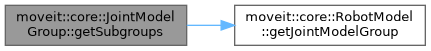Here is the caller graph for this function: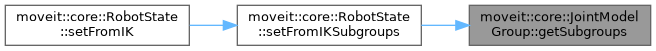const std::vector& moveit::core::JointModelGroup::getUpdatedLinkModelNames ( ) const
inline

Definition at line 246 of file joint_model_group.h.

Here is the caller graph for this function:const std::vector& moveit::core::JointModelGroup::getUpdatedLinkModels ( ) const
inline

Get the names of the links that are to be updated when the state of this group changes. This includes links that are in the kinematic model but outside this group, if those links are descendants of joints in this group that have their values updated. The order is the correct order for updating the corresponding states.

Definition at line 234 of file joint_model_group.h.

Here is the caller graph for this function:const std::set& moveit::core::JointModelGroup::getUpdatedLinkModelsSet ( ) const
inline

Return the same data as getUpdatedLinkModels() but as a set.

Definition at line 240 of file joint_model_group.h.

 const std::vector& moveit::core::JointModelGroup::getUpdatedLinkModelsWithGeometry ( ) const
inline

Get the names of the links that are to be updated when the state of this group changes. This includes links that are in the kinematic model but outside this group, if those links are descendants of joints in this group that have their values updated.

Definition at line 254 of file joint_model_group.h.

 const std::vector& moveit::core::JointModelGroup::getUpdatedLinkModelsWithGeometryNames ( ) const
inline

Definition at line 266 of file joint_model_group.h.

 const std::set& moveit::core::JointModelGroup::getUpdatedLinkModelsWithGeometryNamesSet ( ) const
inline

Definition at line 272 of file joint_model_group.h.

 const std::set& moveit::core::JointModelGroup::getUpdatedLinkModelsWithGeometrySet ( ) const
inline

Return the same data as getUpdatedLinkModelsWithGeometry() but as a set.

Definition at line 260 of file joint_model_group.h.

## ◆ getVariableCount()

 unsigned int moveit::core::JointModelGroup::getVariableCount ( ) const
inline

Get the number of variables that describe this joint group. This includes variables necessary for mimic joints, so will always be >= the number of items returned by getActiveVariableNames()

Definition at line 410 of file joint_model_group.h.

Here is the caller graph for this function: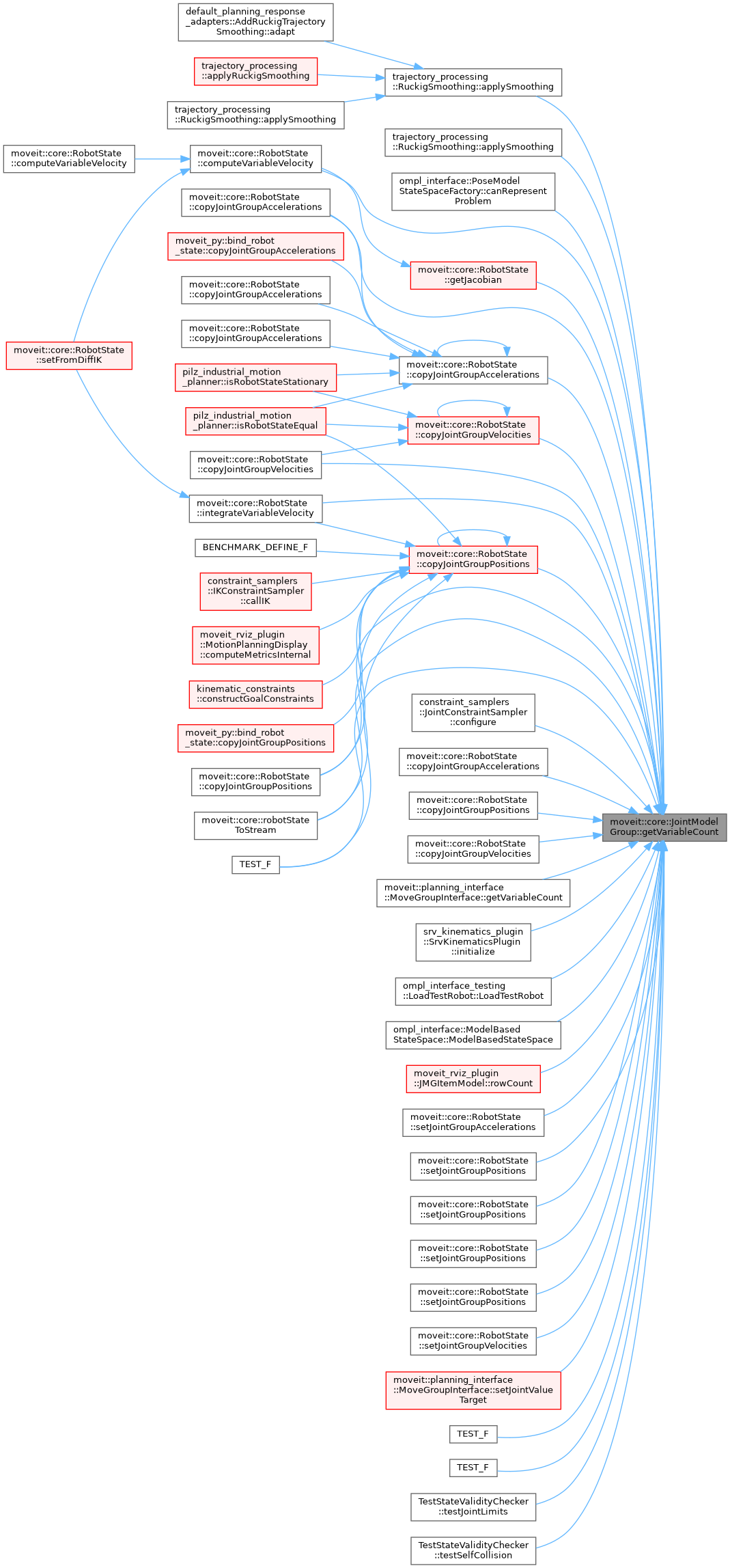## ◆ getVariableDefaultPositions() [1/4]

 bool moveit::core::JointModelGroup::getVariableDefaultPositions ( const std::string & name, std::map< std::string, double > & values ) const

Get the values that correspond to a named state as read from the URDF. Return false on failure.

Definition at line 489 of file joint_model_group.cpp.

Here is the caller graph for this function: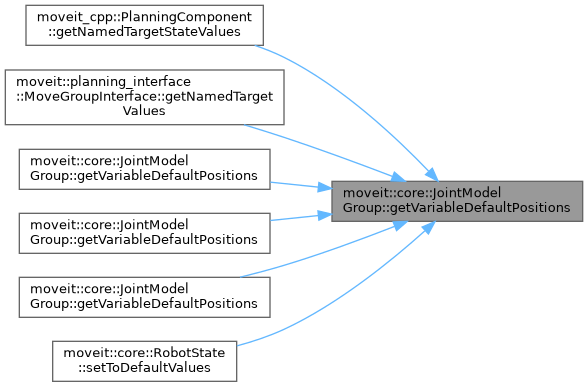## ◆ getVariableDefaultPositions() [2/4]

 void moveit::core::JointModelGroup::getVariableDefaultPositions ( double * values ) const

Compute the default values for the joint group.

Definition at line 498 of file joint_model_group.cpp.

Here is the call graph for this function: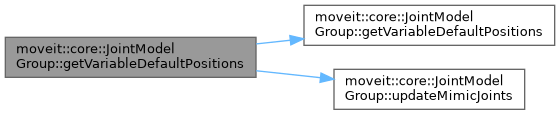## ◆ getVariableDefaultPositions() [3/4]

 void moveit::core::JointModelGroup::getVariableDefaultPositions ( std::map< std::string, double > & values ) const

Compute the default values for the joint group.

Definition at line 505 of file joint_model_group.cpp.

Here is the call graph for this function: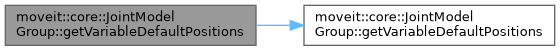## ◆ getVariableDefaultPositions() [4/4]

 void moveit::core::JointModelGroup::getVariableDefaultPositions ( std::vector< double > & values ) const
inline

Compute the default values for the joint group.

Definition at line 309 of file joint_model_group.h.

Here is the call graph for this function:## ◆ getVariableGroupIndex()

 int moveit::core::JointModelGroup::getVariableGroupIndex ( const std::string & variable ) const

Get the index of a variable within the group. Return -1 on error.

Definition at line 589 of file joint_model_group.cpp.

Here is the caller graph for this function: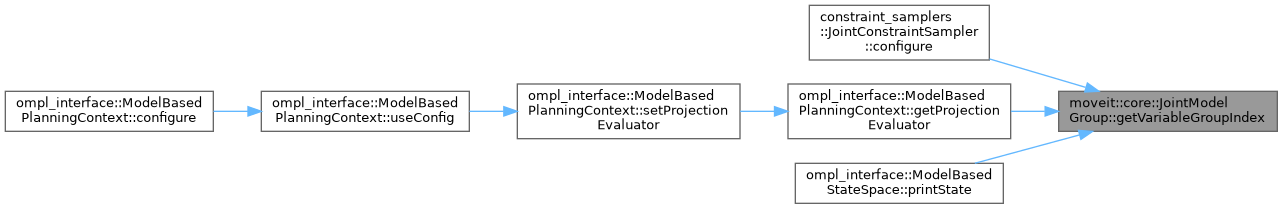## ◆ getVariableIndexList()

 const std::vector& moveit::core::JointModelGroup::getVariableIndexList ( ) const
inline

Get the index locations in the complete robot state for all the variables in this group.

Definition at line 286 of file joint_model_group.h.

Here is the caller graph for this function:## ◆ getVariableNames()

 const std::vector& moveit::core::JointModelGroup::getVariableNames ( ) const
inline

Get the names of the variables that make up the joints included in this group. The number of returned elements is always equal to getVariableCount(). This includes mimic joints.

Definition at line 189 of file joint_model_group.h.

Here is the caller graph for this function:## ◆ getVariableRandomPositions() [1/3]

 void moveit::core::JointModelGroup::getVariableRandomPositions ( random_numbers::RandomNumberGenerator & rng, double * values ) const
inline

Compute random values for the state of the joint group.

Definition at line 319 of file joint_model_group.h.

Here is the caller graph for this function: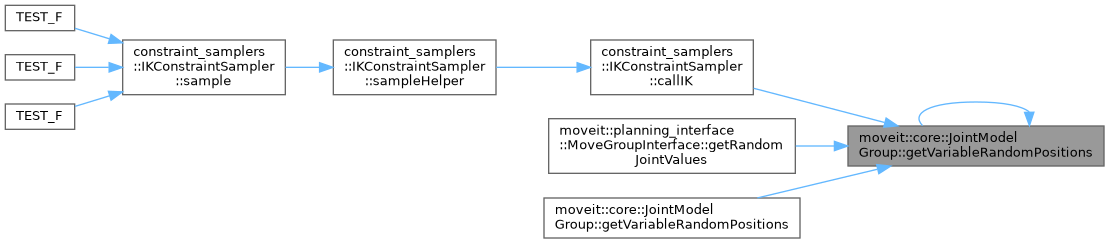## ◆ getVariableRandomPositions() [2/3]

 void moveit::core::JointModelGroup::getVariableRandomPositions ( random_numbers::RandomNumberGenerator & rng, double * values, const JointBoundsVector & active_joint_bounds ) const

Definition at line 336 of file joint_model_group.cpp.

Here is the call graph for this function: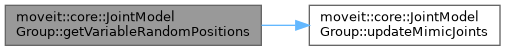## ◆ getVariableRandomPositions() [3/3]

 void moveit::core::JointModelGroup::getVariableRandomPositions ( random_numbers::RandomNumberGenerator & rng, std::vector< double > & values ) const
inline

Compute random values for the state of the joint group.

Definition at line 325 of file joint_model_group.h.

Here is the call graph for this function: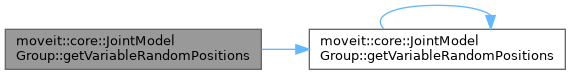## ◆ getVariableRandomPositionsNearBy() [1/8]

 void moveit::core::JointModelGroup::getVariableRandomPositionsNearBy ( random_numbers::RandomNumberGenerator & rng, double * values, const double * near, const double distance ) const
inline

Compute random values for the state of the joint group.

Definition at line 332 of file joint_model_group.h.

Here is the call graph for this function: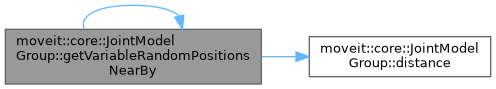Here is the caller graph for this function: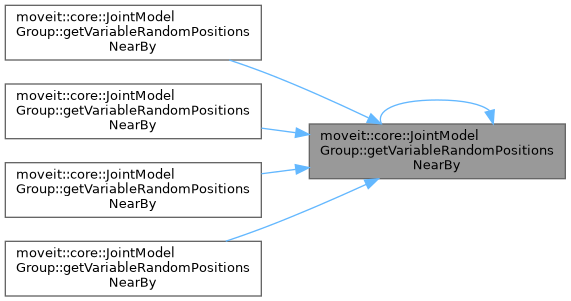## ◆ getVariableRandomPositionsNearBy() [2/8]

 void moveit::core::JointModelGroup::getVariableRandomPositionsNearBy ( random_numbers::RandomNumberGenerator & rng, double * values, const double * near, const std::vector< double > & distances ) const
inline

Compute random values for the state of the joint group.

Definition at line 355 of file joint_model_group.h.

Here is the call graph for this function: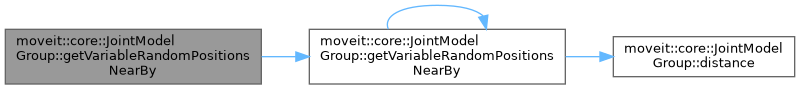## ◆ getVariableRandomPositionsNearBy() [3/8]

 void moveit::core::JointModelGroup::getVariableRandomPositionsNearBy ( random_numbers::RandomNumberGenerator & rng, double * values, const JointBoundsVector & active_joint_bounds, const double * near, const double distance ) const

Compute random values for the state of the joint group.

Definition at line 349 of file joint_model_group.cpp.

Here is the call graph for this function: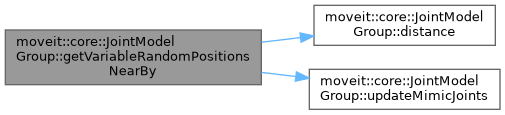## ◆ getVariableRandomPositionsNearBy() [4/8]

 void moveit::core::JointModelGroup::getVariableRandomPositionsNearBy ( random_numbers::RandomNumberGenerator & rng, double * values, const JointBoundsVector & active_joint_bounds, const double * near, const std::map< JointModel::JointType, double > & distance_map ) const

Compute random values for the state of the joint group.

Definition at line 364 of file joint_model_group.cpp.

Here is the call graph for this function: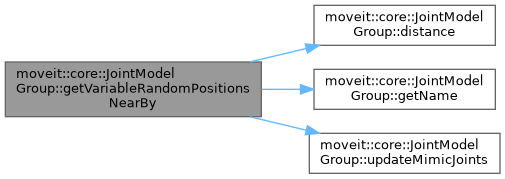## ◆ getVariableRandomPositionsNearBy() [5/8]

 void moveit::core::JointModelGroup::getVariableRandomPositionsNearBy ( random_numbers::RandomNumberGenerator & rng, double * values, const JointBoundsVector & active_joint_bounds, const double * near, const std::vector< double > & distances ) const

Compute random values for the state of the joint group.

Definition at line 390 of file joint_model_group.cpp.

Here is the call graph for this function: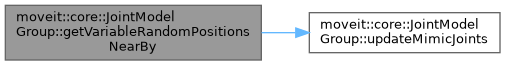## ◆ getVariableRandomPositionsNearBy() [6/8]

 void moveit::core::JointModelGroup::getVariableRandomPositionsNearBy ( random_numbers::RandomNumberGenerator & rng, std::vector< double > & values, const std::vector< double > & near, const std::map< JointModel::JointType, double > & distance_map ) const
inline

Compute random values for the state of the joint group.

Definition at line 346 of file joint_model_group.h.

Here is the call graph for this function:## ◆ getVariableRandomPositionsNearBy() [7/8]

 void moveit::core::JointModelGroup::getVariableRandomPositionsNearBy ( random_numbers::RandomNumberGenerator & rng, std::vector< double > & values, const std::vector< double > & near, const std::vector< double > & distances ) const
inline

Compute random values for the state of the joint group.

Definition at line 361 of file joint_model_group.h.

Here is the call graph for this function:## ◆ getVariableRandomPositionsNearBy() [8/8]

 void moveit::core::JointModelGroup::getVariableRandomPositionsNearBy ( random_numbers::RandomNumberGenerator & rng, std::vector< double > & values, const std::vector< double > & near, double distance ) const
inline

Compute random values for the state of the joint group.

Definition at line 338 of file joint_model_group.h.

Here is the call graph for this function: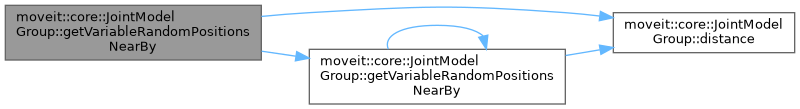## ◆ hasJointModel()

 bool moveit::core::JointModelGroup::hasJointModel ( const std::string & joint ) const

Check if a joint is part of this group.

Definition at line 304 of file joint_model_group.cpp.

Here is the caller graph for this function: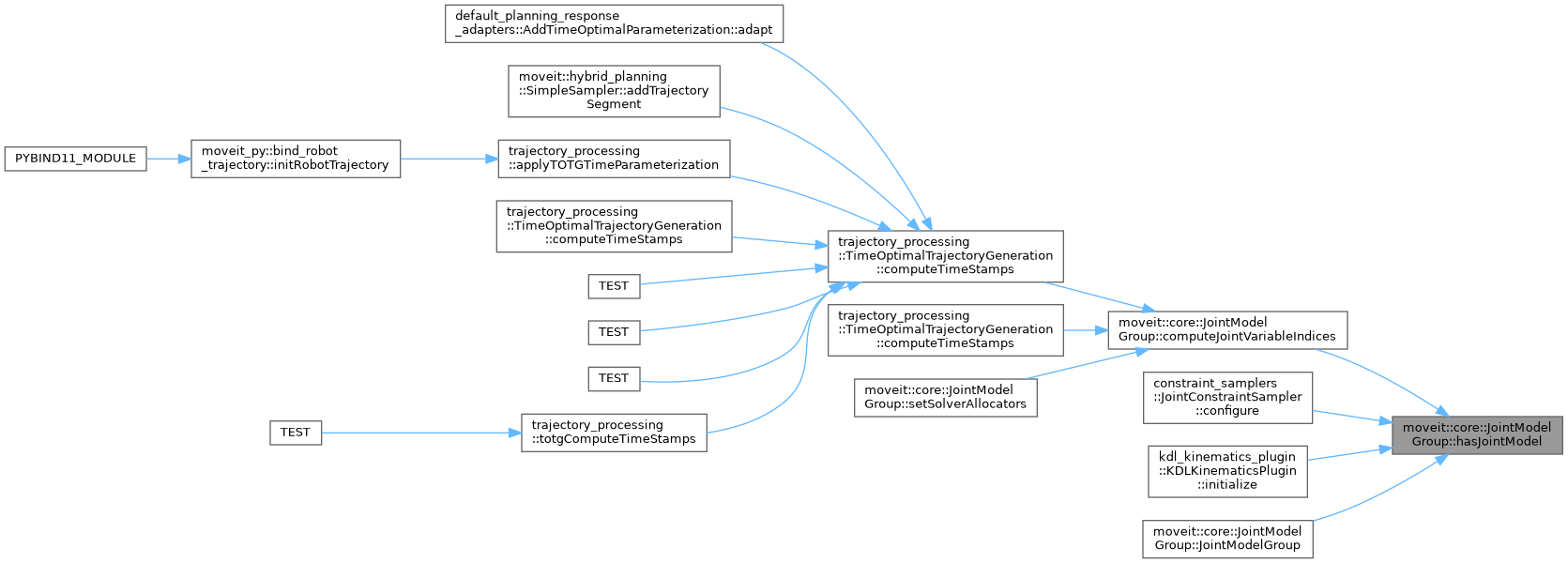Check if a link is part of this group.

Definition at line 309 of file joint_model_group.cpp.

Here is the caller graph for this function: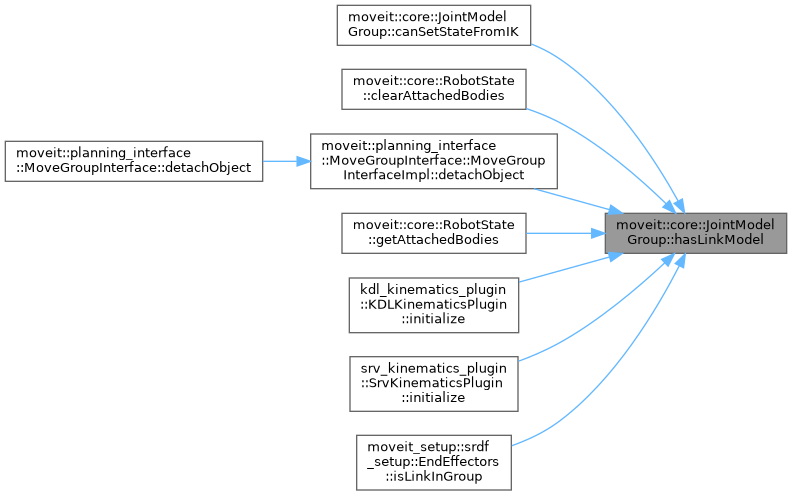## ◆ interpolate()

 void moveit::core::JointModelGroup::interpolate ( const double * from, const double * to, double t, double * state ) const

Definition at line 462 of file joint_model_group.cpp.

Here is the call graph for this function: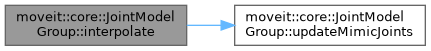## ◆ isChain()

 bool moveit::core::JointModelGroup::isChain ( ) const
inline

Check if this group is a linear chain.

Definition at line 441 of file joint_model_group.h.

Here is the caller graph for this function: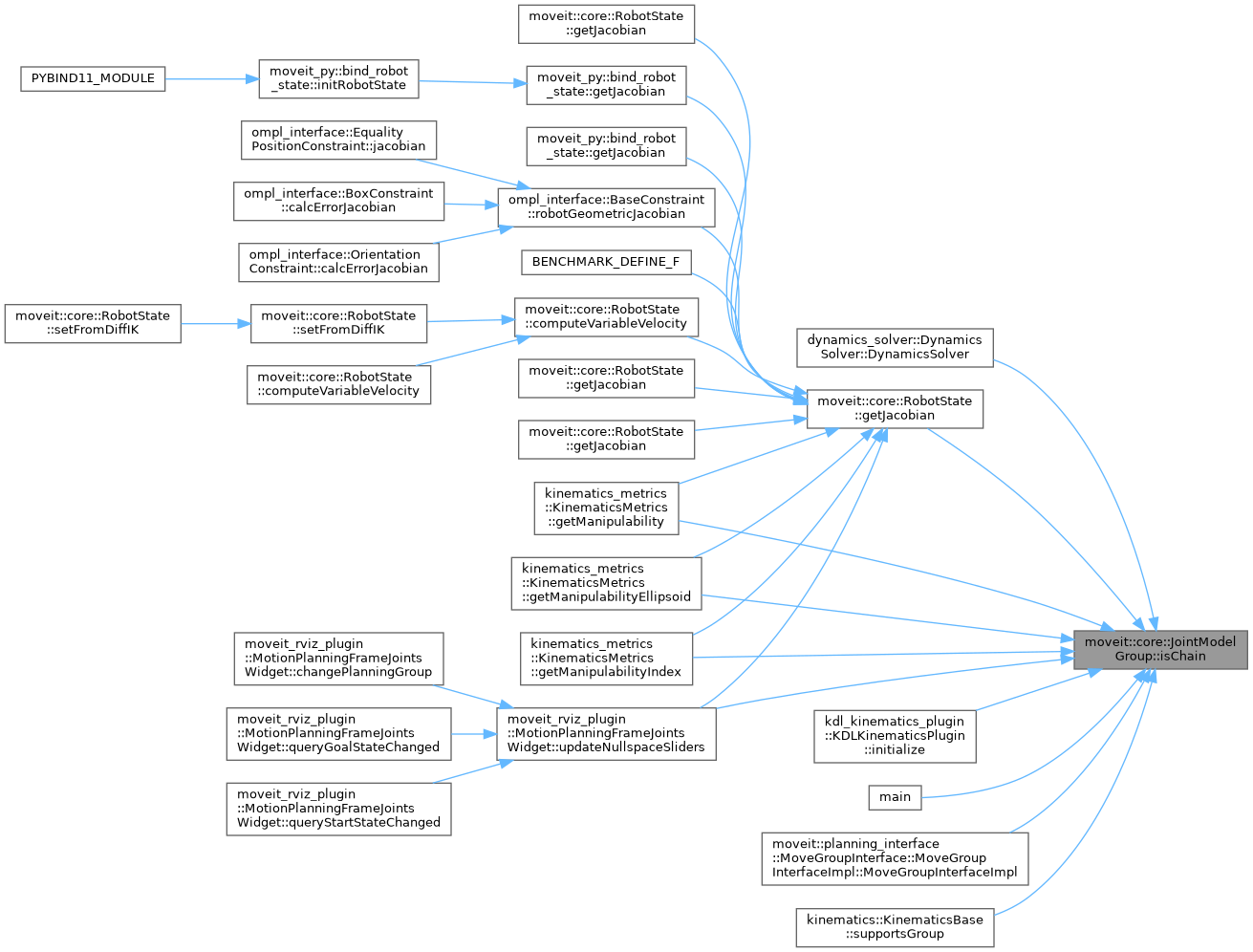## ◆ isContiguousWithinState()

 bool moveit::core::JointModelGroup::isContiguousWithinState ( ) const
inline

Definition at line 458 of file joint_model_group.h.

## ◆ isEndEffector()

 bool moveit::core::JointModelGroup::isEndEffector ( ) const
inline

Check if this group was designated as an end-effector in the SRDF.

Definition at line 453 of file joint_model_group.h.

 bool moveit::core::JointModelGroup::isLinkUpdated ( const std::string & name ) const
inline

True if this name is in the set of links that are to be updated when the state of this group changes. This includes links that are in the kinematic model but outside this group, if those links are descendants of joints in this group that have their values updated.

Definition at line 280 of file joint_model_group.h.

## ◆ isSingleDOFJoints()

 bool moveit::core::JointModelGroup::isSingleDOFJoints ( ) const
inline

Return true if the group consists only of joints that are single DOF.

Definition at line 447 of file joint_model_group.h.

Here is the caller graph for this function: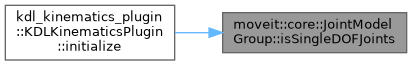## ◆ isSubgroup()

 bool moveit::core::JointModelGroup::isSubgroup ( const std::string & group ) const
inline

Check if the joints of group group are a subset of the joints in this group.

Definition at line 435 of file joint_model_group.h.

Here is the caller graph for this function: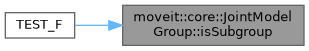## ◆ isValidVelocityMove() [1/2]

 bool moveit::core::JointModelGroup::isValidVelocityMove ( const double * from_joint_pose, const double * to_joint_pose, std::size_t array_size, double dt ) const

Check that the time to move between two waypoints is sufficient given velocity limits.

Definition at line 791 of file joint_model_group.cpp.

Here is the call graph for this function: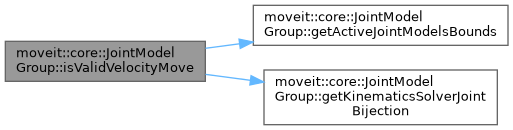## ◆ isValidVelocityMove() [2/2]

 bool moveit::core::JointModelGroup::isValidVelocityMove ( const std::vector< double > & from_joint_pose, const std::vector< double > & to_joint_pose, double dt ) const

Check that the time to move between two waypoints is sufficient given velocity limits.

Definition at line 778 of file joint_model_group.cpp.

## ◆ printGroupInfo()

 void moveit::core::JointModelGroup::printGroupInfo ( std::ostream & out = `std::cout` ) const

Print information about the constructed model.

Definition at line 715 of file joint_model_group.cpp.

Here is the call graph for this function: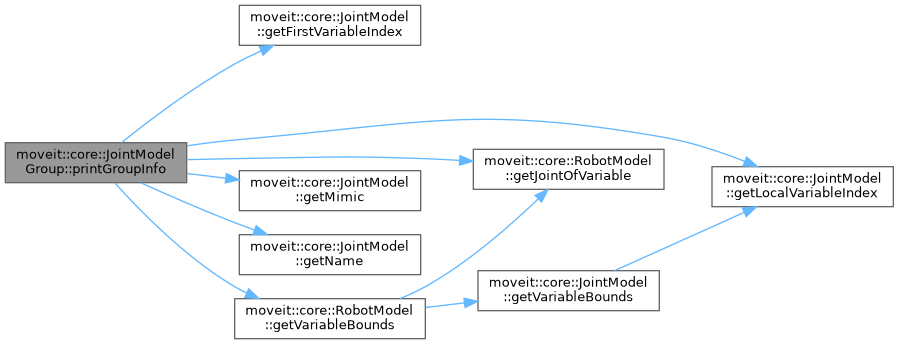## ◆ satisfiesPositionBounds() [1/2]

 bool moveit::core::JointModelGroup::satisfiesPositionBounds ( const double * state, const JointBoundsVector & active_joint_bounds, double margin = `0.0` ) const

Definition at line 411 of file joint_model_group.cpp.

Here is the call graph for this function: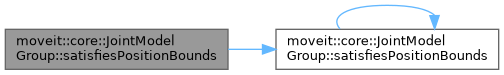## ◆ satisfiesPositionBounds() [2/2]

 bool moveit::core::JointModelGroup::satisfiesPositionBounds ( const double * state, double margin = `0.0` ) const
inline

Definition at line 392 of file joint_model_group.h.

Here is the caller graph for this function: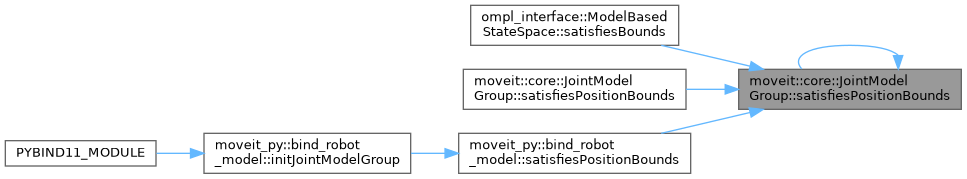## ◆ setDefaultIKTimeout()

 void moveit::core::JointModelGroup::setDefaultIKTimeout ( double ik_timeout )

Set the default IK timeout.

Definition at line 600 of file joint_model_group.cpp.

Here is the caller graph for this function: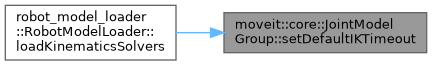## ◆ setEndEffectorName()

 void moveit::core::JointModelGroup::setEndEffectorName ( const std::string & name )

Set the name of the end-effector, and remember this group is indeed an end-effector.

Definition at line 513 of file joint_model_group.cpp.

## ◆ setEndEffectorParent()

 void moveit::core::JointModelGroup::setEndEffectorParent ( const std::string & group, const std::string & link )

If this group is an end-effector, specify the parent group (e.g., the arm holding the eef) and the link the end effector connects to.

Definition at line 518 of file joint_model_group.cpp.

## ◆ setRedundantJoints()

 bool moveit::core::JointModelGroup::setRedundantJoints ( const std::vector< std::string > & joints )
inline

Definition at line 549 of file joint_model_group.h.

## ◆ setSolverAllocators() [1/2]

 void moveit::core::JointModelGroup::setSolverAllocators ( const SolverAllocatorFn & solver, const SolverAllocatorMapFn & solver_map = `SolverAllocatorMapFn()` )
inline

Definition at line 529 of file joint_model_group.h.

Here is the caller graph for this function: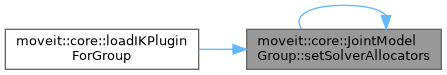## ◆ setSolverAllocators() [2/2]

 void moveit::core::JointModelGroup::setSolverAllocators ( const std::pair< SolverAllocatorFn, SolverAllocatorMapFn > & solvers )

Definition at line 634 of file joint_model_group.cpp.

Here is the call graph for this function: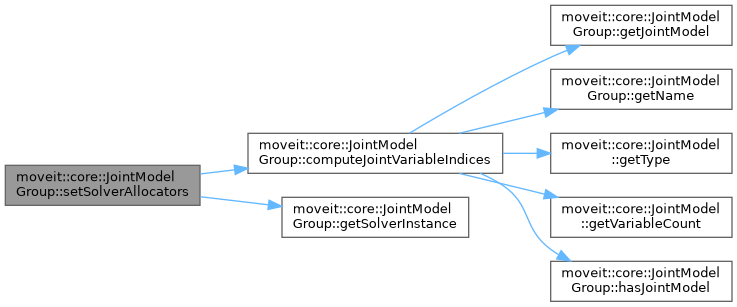## ◆ setSubgroupNames()

 void moveit::core::JointModelGroup::setSubgroupNames ( const std::vector< std::string > & subgroups )

Set the names of the subgroups for this group.

Definition at line 289 of file joint_model_group.cpp.

## ◆ updateMimicJoints()

 void moveit::core::JointModelGroup::updateMimicJoints ( double * values ) const
protected

Update the variable values for the state of a group with respect to the mimic joints. This only updates mimic joints that have the parent in this group. If there is a joint mimicking one that is outside the group, there are no values to be read (values is only the group state)

Definition at line 476 of file joint_model_group.cpp.

Here is the caller graph for this function: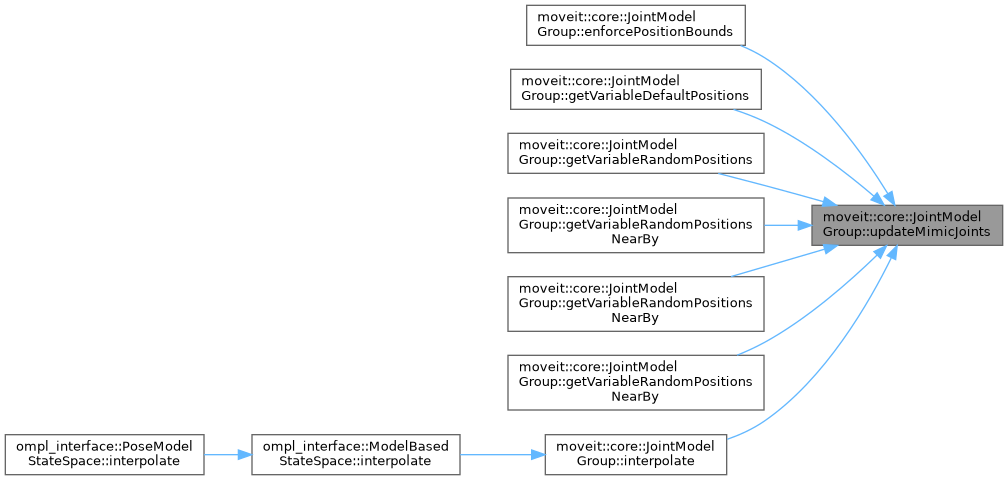## ◆ active_joint_model_name_vector_

 std::vector moveit::core::JointModelGroup::active_joint_model_name_vector_
protected

Names of active joints in the order they appear in the group state.

Definition at line 611 of file joint_model_group.h.

## ◆ active_joint_model_start_index_

 std::vector moveit::core::JointModelGroup::active_joint_model_start_index_
protected

For each active joint model in this group, hold the index at which the corresponding joint state starts in the group state.

Definition at line 653 of file joint_model_group.h.

## ◆ active_joint_model_vector_

 std::vector moveit::core::JointModelGroup::active_joint_model_vector_
protected

Active joint instances in the order they appear in the group state.

Definition at line 608 of file joint_model_group.h.

## ◆ active_joint_models_bounds_

 JointBoundsVector moveit::core::JointModelGroup::active_joint_models_bounds_
protected

The bounds for all the active joint models.

Definition at line 645 of file joint_model_group.h.

## ◆ active_variable_count_

 unsigned int moveit::core::JointModelGroup::active_variable_count_
protected

The number of variables necessary to describe the active joints in this group of joints.

Definition at line 707 of file joint_model_group.h.

## ◆ attached_end_effector_names_

 std::vector moveit::core::JointModelGroup::attached_end_effector_names_
protected

If an end-effector is attached to this group, the name of that end-effector is stored in this variable.

Definition at line 720 of file joint_model_group.h.

## ◆ common_root_

 const JointModel* moveit::core::JointModelGroup::common_root_
protected

The joint that is a common root for all joints in this group (not necessarily part of this group)

Definition at line 637 of file joint_model_group.h.

## ◆ config_

 srdf::Model::Group moveit::core::JointModelGroup::config_
protected

Definition at line 749 of file joint_model_group.h.

## ◆ continuous_joint_model_vector_

 std::vector moveit::core::JointModelGroup::continuous_joint_model_vector_
protected

The set of continuous joints this group contains.

Definition at line 620 of file joint_model_group.h.

## ◆ default_states_

 std::map > moveit::core::JointModelGroup::default_states_
protected

The set of default states specified for this group in the SRDF.

Definition at line 752 of file joint_model_group.h.

## ◆ default_states_names_

 std::vector moveit::core::JointModelGroup::default_states_names_
protected

The names of the default states specified for this group in the SRDF.

Definition at line 755 of file joint_model_group.h.

## ◆ end_effector_name_

 std::string moveit::core::JointModelGroup::end_effector_name_
protected

The name of the end effector, if this group is an end-effector.

Definition at line 728 of file joint_model_group.h.

## ◆ end_effector_parent_

 std::pair moveit::core::JointModelGroup::end_effector_parent_
protected

First: name of the group that is parent to this end-effector group; Second: the link this in the parent group that this group attaches to.

Definition at line 725 of file joint_model_group.h.

## ◆ fixed_joints_

 std::vector moveit::core::JointModelGroup::fixed_joints_
protected

The joints that have no DOF (fixed)

Definition at line 614 of file joint_model_group.h.

## ◆ group_kinematics_

 std::pair moveit::core::JointModelGroup::group_kinematics_
protected

Definition at line 747 of file joint_model_group.h.

## ◆ group_mimic_update_

 std::vector moveit::core::JointModelGroup::group_mimic_update_
protected

Definition at line 745 of file joint_model_group.h.

## ◆ is_chain_

 bool moveit::core::JointModelGroup::is_chain_
protected

Definition at line 730 of file joint_model_group.h.

## ◆ is_contiguous_index_list_

 bool moveit::core::JointModelGroup::is_contiguous_index_list_
protected

True if the state of this group is contiguous within the full robot state; this also means that the index values in variable_index_list_ are consecutive integers.

Definition at line 711 of file joint_model_group.h.

## ◆ is_single_dof_

 bool moveit::core::JointModelGroup::is_single_dof_
protected

Definition at line 732 of file joint_model_group.h.

## ◆ joint_model_map_

 JointModelMapConst moveit::core::JointModelGroup::joint_model_map_
protected

A map from joint names to their instances. This includes all joints in the group.

Definition at line 631 of file joint_model_group.h.

## ◆ joint_model_name_vector_

 std::vector moveit::core::JointModelGroup::joint_model_name_vector_
protected

Names of joints in the order they appear in the group state.

Definition at line 605 of file joint_model_group.h.

## ◆ joint_model_vector_

 std::vector moveit::core::JointModelGroup::joint_model_vector_
protected

Joint instances in the order they appear in the group state.

Definition at line 602 of file joint_model_group.h.

## ◆ joint_roots_

 std::vector moveit::core::JointModelGroup::joint_roots_
protected

The list of active joint models that are roots in this group.

Definition at line 634 of file joint_model_group.h.

## ◆ joint_variables_index_map_

 VariableIndexMap moveit::core::JointModelGroup::joint_variables_index_map_
protected

The group includes all the joint variables that make up the joints the group consists of. This map gives the position in the state vector of the group for each of these variables. Additionally, it includes the names of the joints and the index for the first variable of that joint.

Definition at line 642 of file joint_model_group.h.

protected

A map from link names to their instances.

Definition at line 661 of file joint_model_group.h.

protected

The names of the links in this group.

Definition at line 664 of file joint_model_group.h.

protected

The links that are on the direct lineage between joints and joint_roots_, as well as the children of the joint leafs. May not be in any particular order.

Definition at line 658 of file joint_model_group.h.

protected

The names of the links in this group that also have geometry.

Definition at line 669 of file joint_model_group.h.

protected

Definition at line 666 of file joint_model_group.h.

## ◆ mimic_joints_

 std::vector moveit::core::JointModelGroup::mimic_joints_
protected

Joints that mimic other joints.

Definition at line 617 of file joint_model_group.h.

## ◆ name_

 std::string moveit::core::JointModelGroup::name_
protected

Name of group.

Definition at line 599 of file joint_model_group.h.

## ◆ parent_model_

 const RobotModel* moveit::core::JointModelGroup::parent_model_
protected

Owner model.

Definition at line 596 of file joint_model_group.h.

## ◆ subgroup_names_

 std::vector moveit::core::JointModelGroup::subgroup_names_
protected

The set of labelled subgroups that are included in this group.

Definition at line 714 of file joint_model_group.h.

## ◆ subgroup_names_set_

 std::set moveit::core::JointModelGroup::subgroup_names_set_
protected

The set of labelled subgroups that are included in this group.

Definition at line 717 of file joint_model_group.h.

protected

The list of downstream link names in the order they should be updated (may include links that are not in this group)

Definition at line 685 of file joint_model_group.h.

protected

The list of downstream link names in the order they should be updated (may include links that are not in this group)

Definition at line 681 of file joint_model_group.h.

protected

The list of downstream link models in the order they should be updated (may include links that are not in this group)

Definition at line 677 of file joint_model_group.h.

protected

The list of downstream link models in the order they should be updated (may include links that are not in this group)

Definition at line 673 of file joint_model_group.h.

protected

The list of downstream link names in the order they should be updated (may include links that are not in this group)

Definition at line 701 of file joint_model_group.h.

protected

The list of downstream link names in the order they should be updated (may include links that are not in this group)

Definition at line 697 of file joint_model_group.h.

protected

The list of downstream link models in the order they should be updated (may include links that are not in this group)

Definition at line 693 of file joint_model_group.h.

protected

The list of downstream link models in the order they should be updated (may include links that are not in this group)

Definition at line 689 of file joint_model_group.h.

## ◆ variable_count_

 unsigned int moveit::core::JointModelGroup::variable_count_
protected

The number of variables necessary to describe this group of joints.

Definition at line 704 of file joint_model_group.h.

## ◆ variable_index_list_

 std::vector moveit::core::JointModelGroup::variable_index_list_
protected

The list of index values this group includes, with respect to a full robot state; this includes mimic joints.

Definition at line 649 of file joint_model_group.h.

## ◆ variable_names_

 std::vector moveit::core::JointModelGroup::variable_names_
protected

The names of the DOF that make up this group (this is just a sequence of joint variable names; not necessarily joint names!)

Definition at line 624 of file joint_model_group.h.

## ◆ variable_names_set_

 std::set moveit::core::JointModelGroup::variable_names_set_
protected

The names of the DOF that make up this group (this is just a sequence of joint variable names; not necessarily joint names!)

Definition at line 628 of file joint_model_group.h.

The documentation for this class was generated from the following files: